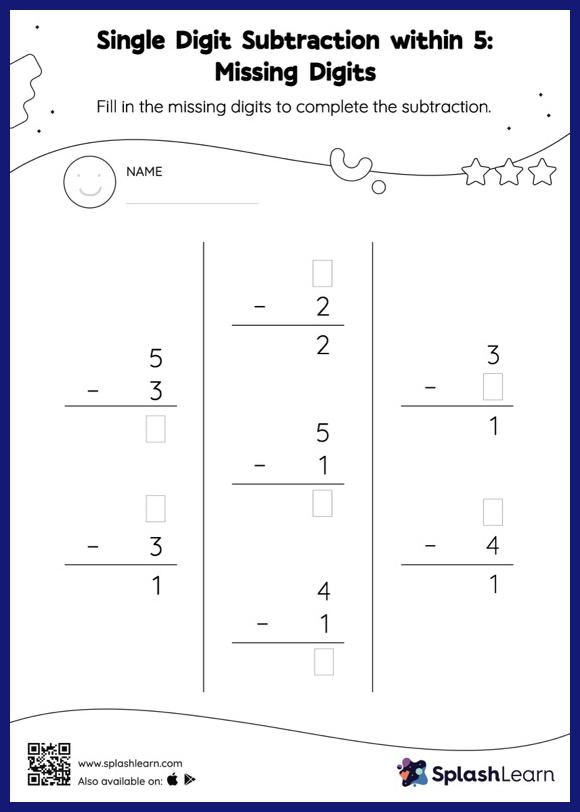# Single Digit Subtraction within 5: Missing Digits Worksheet

Home > Single Digit Subtraction within 5: Missing DigitsChildren use their math skills to complete this single digit subtraction within 5 worksheet. If a minuend or subtrahend is missing, students use the count-back method or the relationship between addition and subtraction to determine the missing number. Also, they subtract the numbers if the missing number is the difference of the subtraction sentence. This single digit subtraction within 5 worksheet offers a tonne of practice with this idea. This worksheet is about practicing the column method in which numbers are written one on top of another. In this method, students use their place value understanding to solve the problems.